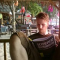Class Central is learner-supported. When you buy through links on our site, we may earn an affiliate commission.

### Overview

##### Class Central Tips
This course aims to help you to draw better statistical inferences from empirical research. First, we will discuss how to correctly interpret p-values, effect sizes, confidence intervals, Bayes Factors, and likelihood ratios, and how these statistics answer different questions you might be interested in. Then, you will learn how to design experiments where the false positive rate is controlled, and how to decide upon the sample size for your study, for example in order to achieve high statistical power. Subsequently, you will learn how to interpret evidence in the scientific literature given widespread publication bias, for example by learning about p-curve analysis. Finally, we will talk about how to do philosophy of science, theory construction, and cumulative science, including how to perform replication studies, why and how to pre-register your experiment, and how to share your results following Open Science principles.

In practical, hands on assignments, you will learn how to simulate t-tests to learn which p-values you can expect, calculate likelihood ratio's and get an introduction the binomial Bayesian statistics, and learn about the positive predictive value which expresses the probability published research findings are true. We will experience the problems with optional stopping and learn how to prevent these problems by using sequential analyses. You will calculate effect sizes, see how confidence intervals work through simulations, and practice doing a-priori power analyses. Finally, you will learn how to examine whether the null hypothesis is true using equivalence testing and Bayesian statistics, and how to pre-register a study, and share your data on the Open Science Framework.

All videos now have Chinese subtitles. More than 30.000 learners have enrolled so far!

If you enjoyed this course, I can recommend following it up with me new course "Improving Your Statistical Questions"

### Syllabus

• Introduction + Frequentist Statistics
• Likelihoods & Bayesian Statistics
• Multiple Comparisons, Statistical Power, Pre-Registration
• Effect Sizes
• Confidence Intervals, Sample Size Justification, P-Curve analysis
• Philosophy of Science & Theory
• Open Science
• Final Exam
• This module contains a practice exam and a graded exam. Both quizzes cover content from the entire course. We recommend making these exams only after you went through all the other modules.

Daniel Lakens

## Reviews

5.0 rating, based on 1 Class Central review

4.9 rating at Coursera based on 750 ratings

•